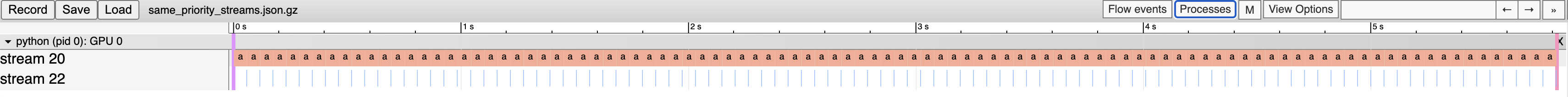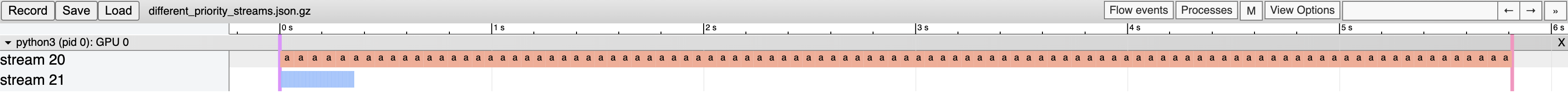The key difference between non_blocking_streams and blocking_streams functions is that the former sets non_blocking to True whereas the latter sets non_blocking to False.

with torch.cuda.stream(first_stream):
for i in range(len(matrix_on_gpu)):
torch.matmul(matrix_on_gpu[i], matrix_on_gpu[i])

with torch.cuda.stream(second_stream):
for i in range(len(data_on_gpu)):
data_on_gpu[i].to(cpu, non_blocking=True)

with torch.cuda.stream(third_stream):
for i in range(len(data_on_cpu)):
data_on_cpu[i].to(cuda, non_blocking=True)


In both functions, first_stream executes computation kernels and second_stream transfers data from the device to the host. When non_blocking is False, second_stream does a synchronization after each iteration of the for loop. The cudaStreamSynchronize calls in the trace show this. These calls block the CPU from moving forward until the kernel execution is complete. On the other hand when non_blocking is True kernels on third_stream can be scheduled and launched while kernels are still executing on second_stream. Thus, all three streams overlap in the non_blocking_streams function whereas only first_stream and second_stream overlap in the blocking_streams function.

## Discussion

A precise measurement of the time spent by memory/computation kernels can be calculated using Holistic Trace Analysis (HTA). The image below shows that the overlap of memory and computation kernels is 32.3% and 16.5% in the non_blocking_streams and blocking_streams functions respectively.

Additionally, HTA also provides a breakdown of the time taken by each kernel.

Here’s a notebook showing how to generate the plots using HTA. Each function was profiled individually and had its unique trace file when analyzed with HTA.

Which operations can run concurrently on the GPU with one another across different streams?

• Computation on the host
• Computation on the device
• Memory transfers from the host to the device
• Memory transfers from the device to the host
• Memory transfers within the memory of a device
• Memory transfers among devices

Which operations are asynchronous with respect to the host?

• Kernel launches
• Memory copies within a single device’s memory
• Memory copy from host to device of a memory block if 64KB or less
• Memory copies performed by functions that are suffixed with Async (e.g. cudaMemcpyAsync, cudaMemcpyPeerAsync, cudaMemsetAsync etc.)
• Memory set function calls (cudaMemset)

How many streams can be launched simultaneously?

At most 128 kernels can run concurrently on the P100, V100, A100 and H100 GPUs. Assuming each kernel is executing on a unique stream, the number of streams that can run concurrently is 128. It is possible to have more than 128 streams in an application. In ML applications, achieving concurrent execution of more than a few streams is difficult to achieve as each kernel should run long enough to achieve overlap and not saturate the GPU memory and CUDA/Tensor cores.

Can data transfers to and from the device be overlapped?

Yes, assuming the GPU has the capability. In particular, the GPU must have asyncEngineCount greater than 0. This value can be checked using the code snippet below:

int deviceCount; cudaGetDeviceCount(&deviceCount);
int device;

for (device = 0; device < deviceCount; ++device) {
cudaGetDeviceProperties(&deviceProp, device);
printf("Number of asynchronous engines %d", deviceProp.asyncEngineCount);
}


Additionally, any host memory involved must be page-locked.

Note: Some OS and CUDA version combinations do not require the host memory to be page locked as can be seen from the trace in this puzzler.

Can data transfer and kernel execution be overlapped?

Yes, assuming the GPU has asyncEngineCount equal to 2. If host memory is involved in the data transfer it must be page-locked.

Note: Some OS and CUDA version combinations do not require the host memory to be page locked as can be seen from the trace in this puzzler.

How can kernels across streams be synchronized?

There are various ways to explicitly synchronize streams with each other.

1. cudaDeviceSynchronize() - waits until all preceding commands in all streams of all host threads have completed.
2. cudaStreamSynchronize() - takes a stream as a parameter and waits until all preceding commands in the given stream have completed. This can be used to synchronize the host with a specific stream allowing other streams to continue executing on the device.
3. cudaStreamWaitEvent() - takes a stream and event as parameters and makes the stream wait for the event to complete.
4. cudaStreamQuery() - provides applications to query an asynchronous stream for completion status.

This table provides a mapping between the PyTorch and CUDA API for the explicit synchronization function calls

CUDA PyTorch
cudaStreamSynchronize torch.cuda.Stream.synchronize
cudaStreamWaitEvent torch.cuda.Stream.wait_event
cudaStreamQuery None

Can streams block each other?

Yes, if any of the following operations is issued in-between them by the host thread:

• Pinned host memory allocation
• Device memory allocation
• Device memory set
• Memory copy between two addresses to the same device memory
• Any CUDA command to the default (a.k.a. NULL) stream
• A switch between the L1/shared memory configurations

In other words, the operations above cause implicit synchronization.

Can computational kernels such as matrix multiplication and vector ops run simultaneously on different streams?

Yes, here’s a code snippet demonstrating the simultaneous matrix multiplication and vector operations.

def streams():
torch.backends.cuda.matmul.allow_tf32 = True
with torch.cuda.stream(first_stream):
for i in range(len(matrix_on_gpu)):
torch.matmul(matrix_on_gpu[i], matrix_on_gpu[i])

with torch.cuda.stream(second_stream):
for i in range(len(matrix_on_gpu)):
torch.pow(matrix_on_gpu[i], 3)

first_stream = torch.cuda.Stream()
second_stream = torch.cuda.Stream()
cuda = torch.device("cuda")

matrix_on_gpu = [torch.rand((1024, 1024), device=cuda) for _ in range(1000)]


Can streams be assigned a priority (as in priority queues)?

Yes, users can prioritize work on a stream by assigning a priority to it. PyTorch allows users to set two levels of priority - low priority (zero) and high priority (minus one). E.g.

s = torch.cuda.Stream(priority = -1)


By default, streams have priority zero, unless specified otherwise.

What is the impact of having different priority on two streams?

Using the streams function from above we show the impact of having the same/different priority, when using two streams.

def same_priority():
with torch.cuda.stream(first_stream):
for i in range(len(matrix_on_gpu)):
torch.matmul(matrix_on_gpu[i], matrix_on_gpu[i])

with torch.cuda.stream(second_stream):
for i in range(len(matrix_on_gpu)):
torch.pow(matrix_on_gpu[i], 3)

def different_priority():
with torch.cuda.stream(first_stream):
for i in range(len(matrix_on_gpu)):
torch.matmul(matrix_on_gpu[i], matrix_on_gpu[i])

with torch.cuda.stream(third_stream):
for i in range(len(matrix_on_gpu)):
torch.pow(matrix_on_gpu[i], 3)

first_stream = torch.cuda.Stream(priority = 0)
second_stream = torch.cuda.Stream(priority = 0)
third_stream = torch.cuda.Stream(priority = -1)

cuda = torch.device("cuda")
matrix_on_gpu = [torch.rand((1024, 1024), device=cuda) for _ in range(1000)]


Profiling the same_priority function we see that the last pow kernel finishes at about 5.8 seconds. On the other hand, in the different priority function all the pow kernels finish execution in about 350ms. This clearly demonstrates the impact of setting the priority on a stream.Streams with same priorityStreams with different priority

Here are the trace files for the same_priority and different_priority functions.

## What should you remember in years to come?

Streams provide a great mechanism to execute kernels concurrently but watch out for implicit synchronization events as they can be major blockers to achieving concurrency.

## Explore more

Overhead of CUDA Streams in PyTorch